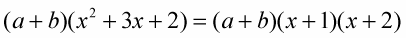##### German All-in-One For DummiesYou can group terms for factoring in expressions where the terms don't share a common factor or common variable. When grouping six terms for factoring, there’s the chance that the groups can be two groups of three terms or three groups of two terms each.

## Dividing terms into two groups of three

Factor this expression by dividing the terms into two groups of three: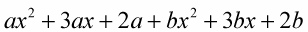1. Look for the common factor in each group.

The first three terms have a common factor of a, and the second three terms have a common factor of b.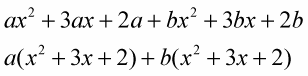2. Factor.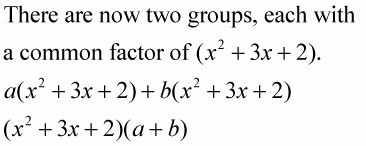3. Factor the quadratic using the unFOIL method.

The first factor is a quadratic.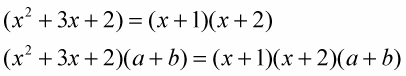## Dividing terms into three groups of two

Grouping the terms two at a time (into three groups) is another way to work the same problem:1. Rearrange the terms, putting the x–squared variables, the x variables, and the numbers together.

You end up with: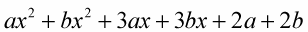2. Look for the common factor in each group, and factor.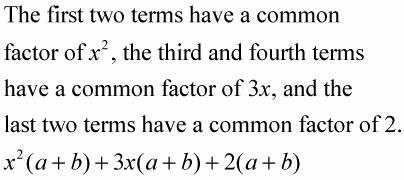3. Factor.

You have three terms, each with a factor of (a + b). Take the (a + b) out of each term to get: